# Wavelet Block Thresholding¶

Important: Please read the installation page for details about how to install the toolboxes. $\newcommand{\dotp}{\langle #1, #2 \rangle}$ $\newcommand{\enscond}{\lbrace #1, #2 \rbrace}$ $\newcommand{\pd}{ \frac{ \partial #1}{\partial #2} }$ $\newcommand{\umin}{\underset{#1}{\min}\;}$ $\newcommand{\umax}{\underset{#1}{\max}\;}$ $\newcommand{\umin}{\underset{#1}{\min}\;}$ $\newcommand{\uargmin}{\underset{#1}{argmin}\;}$ $\newcommand{\norm}{\|#1\|}$ $\newcommand{\abs}{\left|#1\right|}$ $\newcommand{\choice}{ \left\{ \begin{array}{l} #1 \end{array} \right. }$ $\newcommand{\pa}{\left(#1\right)}$ $\newcommand{\diag}{{diag}\left( #1 \right)}$ $\newcommand{\qandq}{\quad\text{and}\quad}$ $\newcommand{\qwhereq}{\quad\text{where}\quad}$ $\newcommand{\qifq}{ \quad \text{if} \quad }$ $\newcommand{\qarrq}{ \quad \Longrightarrow \quad }$ $\newcommand{\ZZ}{\mathbb{Z}}$ $\newcommand{\CC}{\mathbb{C}}$ $\newcommand{\RR}{\mathbb{R}}$ $\newcommand{\EE}{\mathbb{E}}$ $\newcommand{\Zz}{\mathcal{Z}}$ $\newcommand{\Ww}{\mathcal{W}}$ $\newcommand{\Vv}{\mathcal{V}}$ $\newcommand{\Nn}{\mathcal{N}}$ $\newcommand{\NN}{\mathcal{N}}$ $\newcommand{\Hh}{\mathcal{H}}$ $\newcommand{\Bb}{\mathcal{B}}$ $\newcommand{\Ee}{\mathcal{E}}$ $\newcommand{\Cc}{\mathcal{C}}$ $\newcommand{\Gg}{\mathcal{G}}$ $\newcommand{\Ss}{\mathcal{S}}$ $\newcommand{\Pp}{\mathcal{P}}$ $\newcommand{\Ff}{\mathcal{F}}$ $\newcommand{\Xx}{\mathcal{X}}$ $\newcommand{\Mm}{\mathcal{M}}$ $\newcommand{\Ii}{\mathcal{I}}$ $\newcommand{\Dd}{\mathcal{D}}$ $\newcommand{\Ll}{\mathcal{L}}$ $\newcommand{\Tt}{\mathcal{T}}$ $\newcommand{\si}{\sigma}$ $\newcommand{\al}{\alpha}$ $\newcommand{\la}{\lambda}$ $\newcommand{\ga}{\gamma}$ $\newcommand{\Ga}{\Gamma}$ $\newcommand{\La}{\Lambda}$ $\newcommand{\si}{\sigma}$ $\newcommand{\Si}{\Sigma}$ $\newcommand{\be}{\beta}$ $\newcommand{\de}{\delta}$ $\newcommand{\De}{\Delta}$ $\newcommand{\phi}{\varphi}$ $\newcommand{\th}{\theta}$ $\newcommand{\om}{\omega}$ $\newcommand{\Om}{\Omega}$

This numerical tour presents block thresholding methods, that makes use of the structure of wavelet coefficients of natural images to perform denoising. Theoretical properties of block thresholding were investigated in CaiSilv Cai99 HallKerkPic99

In :
using PyPlot
using NtToolBox
arequire("NtToolBox")

WARNING: Base.UTF8String is deprecated, use String instead.
WARNING: Base.UTF8String is deprecated, use String instead.
WARNING: Base.UTF8String is deprecated, use String instead.
WARNING: Base.UTF8String is deprecated, use String instead.
WARNING: Base.UTF8String is deprecated, use String instead.
WARNING: Base.UTF8String is deprecated, use String instead.
likely near In:4
WARNING: Base.UTF8String is deprecated, use String instead.
likely near In:4
WARNING: Base.UTF8String is deprecated, use String instead.
likely near In:4
WARNING: Base.UTF8String is deprecated, use String instead.
likely near In:4
WARNING: Base.UTF8String is deprecated, use String instead.
likely near In:4
WARNING: Base.UTF8String is deprecated, use String instead.
likely near In:4
WARNING: Base.UTF8String is deprecated, use String instead.
likely near In:4
WARNING: Base.UTF8String is deprecated, use String instead.
likely near In:4
WARNING: Base.UTF8String is deprecated, use String instead.
likely near In:4
WARNING: Base.UTF8String is deprecated, use String instead.
likely near In:4
WARNING: Base.UTF8String is deprecated, use String instead.
likely near In:4
WARNING: Base.UTF8String is deprecated, use String instead.
likely near In:4
WARNING: Base.UTF8String is deprecated, use String instead.
likely near In:4
WARNING: Base.UTF8String is deprecated, use String instead.
likely near In:4
WARNING: Base.UTF8String is deprecated, use String instead.
likely near In:4
WARNING: Base.UTF8String is deprecated, use String instead.
likely near In:4
WARNING: Base.UTF8String is deprecated, use String instead.
likely near In:4
WARNING: Base.UTF8String is deprecated, use String instead.
likely near In:4
WARNING: Base.UTF8String is deprecated, use String instead.
likely near In:4
WARNING: Base.UTF8String is deprecated, use String instead.
likely near In:4
WARNING: Base.UTF8String is deprecated, use String instead.
likely near In:4
WARNING: Base.UTF8String is deprecated, use String instead.
likely near In:4
WARNING: Base.UTF8String is deprecated, use String instead.
likely near In:4
WARNING: Base.UTF8String is deprecated, use String instead.
likely near In:4
WARNING: Base.UTF8String is deprecated, use String instead.
likely near In:4
WARNING: Base.UTF8String is deprecated, use String instead.
likely near In:4
WARNING: Base.UTF8String is deprecated, use String instead.
likely near In:4
WARNING: Base.UTF8String is deprecated, use String instead.
likely near In:4
WARNING: Base.UTF8String is deprecated, use String instead.
likely near In:4
WARNING: Base.UTF8String is deprecated, use String instead.
likely near In:4
WARNING: Base.UTF8String is deprecated, use String instead.
likely near In:4
WARNING: Base.UTF8String is deprecated, use String instead.
likely near In:4
WARNING: Base.UTF8String is deprecated, use String instead.
likely near In:4
WARNING: Base.UTF8String is deprecated, use String instead.
likely near In:4
WARNING: Base.UTF8String is deprecated, use String instead.
likely near In:4
WARNING: Base.UTF8String is deprecated, use String instead.
likely near In:4
WARNING: Base.UTF8String is deprecated, use String instead.
likely near In:4
WARNING: Base.UTF8String is deprecated, use String instead.
likely near In:4
WARNING: Base.UTF8String is deprecated, use String instead.
likely near In:4
WARNING: Base.UTF8String is deprecated, use String instead.
likely near In:4
in depwarn(::String, ::Symbol) at .\deprecated.jl:64
in #parse_file#6(::Array{Any,1}, ::Function, ::String) at C:\Users\Ayman\.julia\v0.5\Autoreload\src\files.jl:63
in execute_request(::ZMQ.Socket, ::IJulia.Msg) at C:\Users\Ayman\.julia\v0.5\IJulia\src\execute_request.jl:157
in eventloop(::ZMQ.Socket) at C:\Users\Ayman\.julia\v0.5\IJulia\src\eventloop.jl:8
WARNING: replacing module NtToolBox


## Generating a Noisy Image¶

Here we use an additive Gaussian noise.

Size of the image of $N=n \times n$ pixels.

In :
n = 256;

WARNING: Base.UTF8String is deprecated, use String instead.
likely near C:\Users\Ayman\.julia\v0.5\IJulia\src\kernel.jl:31
WARNING: Base.UTF8String is deprecated, use String instead.
likely near C:\Users\Ayman\.julia\v0.5\IJulia\src\kernel.jl:31
WARNING: Base.UTF8String is deprecated, use String instead.
likely near C:\Users\Ayman\.julia\v0.5\IJulia\src\kernel.jl:31
WARNING: Base.UTF8String is deprecated, use String instead.
likely near C:\Users\Ayman\.julia\v0.5\IJulia\src\kernel.jl:31
WARNING: Base.UTF8String is deprecated, use String instead.
likely near C:\Users\Ayman\.julia\v0.5\IJulia\src\kernel.jl:31
WARNING: Base.UTF8String is deprecated, use String instead.
likely near C:\Users\Ayman\.julia\v0.5\IJulia\src\kernel.jl:31


First we load an image $f_0 \in \RR^N$.

In :
f0 = rescale(load_image("NtToolBox/src/data/boat.png", n));


Display it.

In :
figure(figsize = (5,5))
imageplot(f0)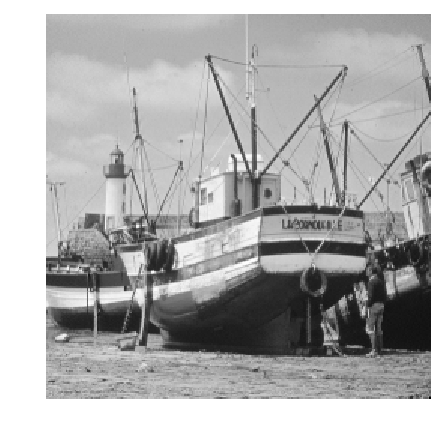Noise level.

In :
sigma = .08;


Generate a noisy image $f=f_0+\epsilon$ where $\epsilon \sim \Nn(0,\si^2\text{Id}_N)$.

In :
using Distributions
f = f0 + sigma.*rand(Normal(), n, n);


Display it.

In :
figure(figsize = (5,5))
imageplot(clamP(f))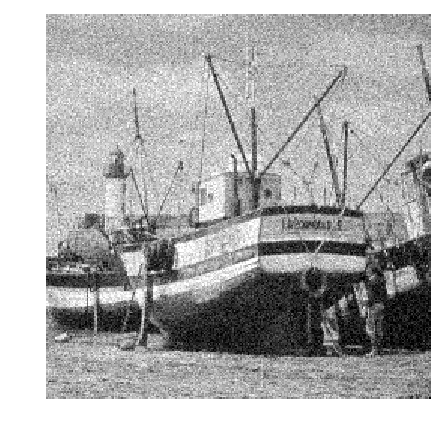## Orthogonal Wavelet Thresholding¶

We first consider the traditional wavelet thresholding method.

Parameters for the orthogonal wavelet transform.

In :
Jmin = 4;


Shortcuts for the foward and backward wavelet transforms.

In :
wav  = f -> NtToolBox.perform_wavelet_transf(f, Jmin, +1)
iwav = fw -> NtToolBox.perform_wavelet_transf(fw, Jmin, -1);


Display the original set of noisy coefficients.

In :
figure(figsize = (10, 10))
plot_wavelet(wav(f), Jmin)
show()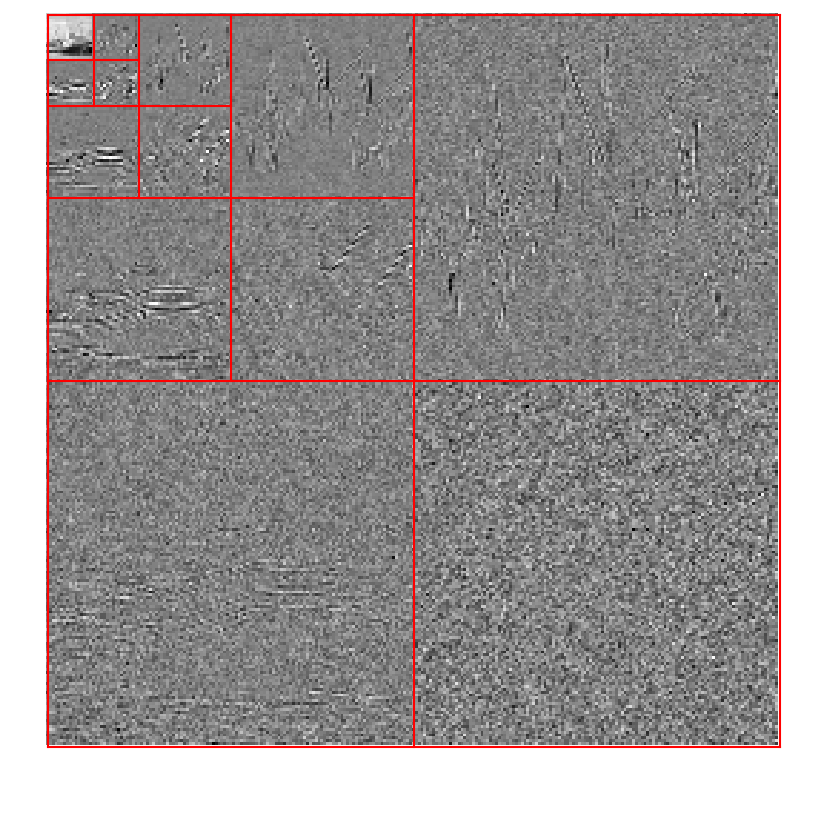Denoting $\Ww$ and $\Ww^*$ the forward and backward wavelet transform, wavelet thresholding $\tilde f$ is defined as

$$\tilde f = \Ww^* \circ \theta_T \circ \Ww(f)$$

where $T>0$ is the threshold, that should be adapted to the noise level.

The thresholding operator is applied component-wise

$$\th_T(x)_i = \psi_T(x_i) x_i$$

where $\psi_T$ is an atenuation fonction. In this tour, we use the James Stein (JS) attenuation:

$$\psi_T(s) = \max\pa{ 0, 1-\frac{T^2}{s^2} }$$
In :
psi = (s, T) -> max(1 - T^2./max(abs(s).^2, 1e-9.*ones(size(s))), zeros(size(s)));


Display the thresholding function $\th_T$.

In :
s = linspace(-3, 3, 1024)

plot(s, s.*psi(s, 1))
plot(s, s, "r--")

show()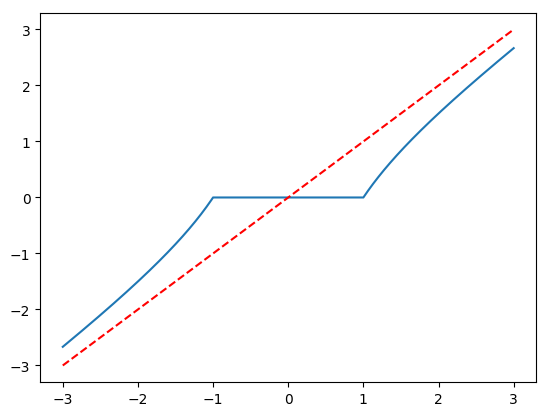Thresholding operator.

In :
theta = (x, T) -> psi(x, T).*x
ThreshWav = (f, T) -> iwav(theta(wav(f), T));


Test the thresholding.

In :
T = 1.5*sigma

figure(figsize = (5, 5))
imageplot(clamP(ThreshWav(f, T)))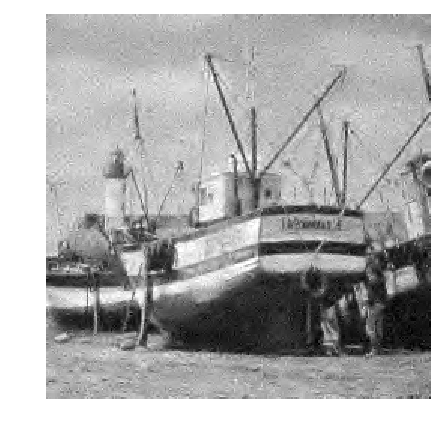Exercise 1

Display the evolution of the denoising SNR when $T$ varies. Store in $f_{Thresh}$ the optimal denoising result.

In :
include("NtSolutions/denoisingwav_4_block/exo1.jl")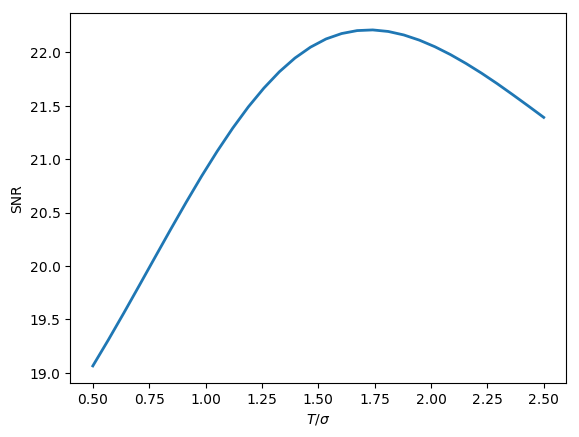Out:
256×256 Array{Float64,2}:
0.625968  0.694511  0.664028  0.685825  …  0.587188  0.58559   0.556904
0.666833  0.713415  0.698709  0.719822     0.588342  0.582681  0.582041
0.687508  0.723447  0.757062  0.723292     0.584483  0.58857   0.618832
0.852152  0.727595  0.629546  0.656954     0.629153  0.629052  0.646233
0.6322    0.617701  0.726676  0.7196       0.673415  0.663018  0.661216
0.568712  0.733649  0.651538  0.684211  …  0.657218  0.644178  0.645722
0.705647  0.644722  0.693751  0.672634     0.630126  0.610606  0.614323
0.682008  0.659418  0.667178  0.680056     0.565555  0.605219  0.578848
0.663885  0.678039  0.67714   0.650876     0.583889  0.570246  0.527736
0.688729  0.649929  0.651663  0.739553     0.592867  0.606361  0.58689
0.715614  0.633788  0.634491  0.801354  …  0.580648  0.553483  0.55032
0.696123  0.666799  0.706528  0.635616     0.535158  0.571715  0.581837
0.685458  0.682204  0.665456  0.725485     0.598165  0.580181  0.576446
⋮                                       ⋱                      ⋮
0.636211  0.488664  0.280411  0.418032     0.528137  0.556917  0.515754
0.569564  0.605694  0.741009  0.75532   …  0.582789  0.527077  0.473523
0.514487  0.604099  0.664932  0.647077     0.512832  0.506804  0.456944
0.631128  0.666075  0.698192  0.704423     0.356548  0.510958  0.480715
0.765495  0.714023  0.667206  0.721657     0.495711  0.436455  0.445046
0.718283  0.718393  0.717137  0.689142     0.561636  0.498873  0.468732
0.757006  0.747529  0.738718  0.753412  …  0.631288  0.560233  0.498495
0.710838  0.714638  0.715273  0.722023     0.598957  0.586513  0.437358
0.672412  0.678607  0.68473   0.693114     0.528807  0.546961  0.507658
0.672463  0.673304  0.689778  0.692533     0.545252  0.493636  0.645247
0.686829  0.671221  0.702466  0.699128     0.529137  0.63748   0.435184
0.643881  0.713313  0.683531  0.696336  …  0.489809  0.560741  0.183504
In :
## Insert your code here.


Display the optimal thresolding.

In :
figure(figsize = (5, 5))
imageplot(clamP(fThresh), @sprintf("SNR = %.1f dB", snr(f0, fThresh)))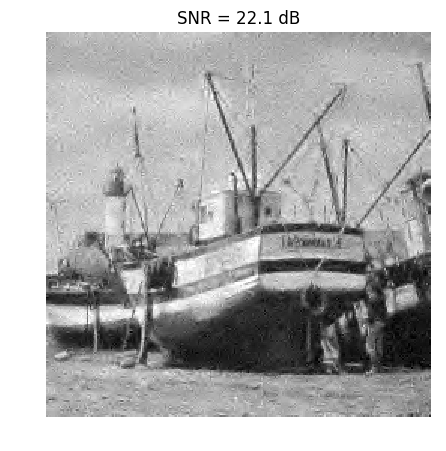Out:
PyObject <matplotlib.text.Text object at 0x00000000292193C8>

## Block Thresholding Operator¶

A block thresholding operator of coefficients $x=(x_i)_{i=1}^P \in \RR^P$ is defined using a partition $B$ into a set of blocks $b$

$$\{1,\ldots,P\} = \bigcup_{b \in B} b.$$

$$\forall i \in b, \quad \theta_T(x)_i = \psi_T\left( \norm{x_b}_2 \right) x_i$$

where $x_b = (x_j)_{j \in B} \in \RR^{\abs{b}}$. One thus thresholds the $\ell^2$ norm (the energy) of each block rather than each coefficient independently.

For image-based thresholding, we use a partition in square blocks of equal size $w \times w$.

The block size $w$.

In :
w = 4
n = 256;


Compute indexing of the blocks.

In :
include("NtToolBox/src/ndgrid.jl")
(X, Y, dX, dY) = ndgrid(1:w:n-w+1, 1:w:n-w+1, 0:w-1, 0:w-1)

I = (X + dX) + (Y + dY - 1).*n
for k in 1:Base.div(n, w)
for l in 1:Base.div(n, w)
I[k, l, :, :] = transpose(I[k, l, :, :])
end
end


Block extraction operator. It returns the set $\{x_b\}_{b \in B}$ of block-partitioned coefficients.

In :
block = x -> x[I];


Block reconstruction operator.

In :
function assign(M,I,H)
M_temp = M
M_temp[I] = H
return reshape(M_temp, n, n)
end

iblock = H -> assign(zeros(n, n), I, H);


Check that block extraction / reconstruction gives perfect reconstruction.

In :
print(string("Should be 0:", string(norm(f - iblock(block(f))))))

Should be 0:0.0

Compute the average energy of each block, and duplicate.

In :
function energy(H)
H_tmp = copy(H)
for i in 1:Base.div(n, w)
for j in 1:Base.div(n, w)
H_tmp[i, j, :, :] = sqrt(mean(H_tmp[i, j, :, :].^2))#*np.ones([1,1])
end
end
return H_tmp
end;


Block thresholding operator.

In :
Thresh = (H, T) -> psi(energy(H), T).*H
ThreshBlock = (x, T) -> iblock(Thresh(block(x), T));


Exercise 2

Test the effect of block thresholding on the image $f_0$ itself, for increasing value of $T$. Of course directly thresholding the image has no interest, this is just to vizualize the effect.

In :
include("NtSolutions/denoisingwav_4_block/exo2.jl")